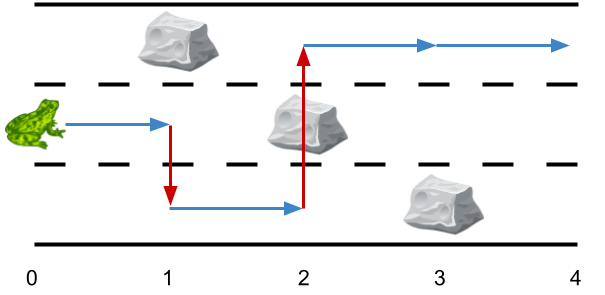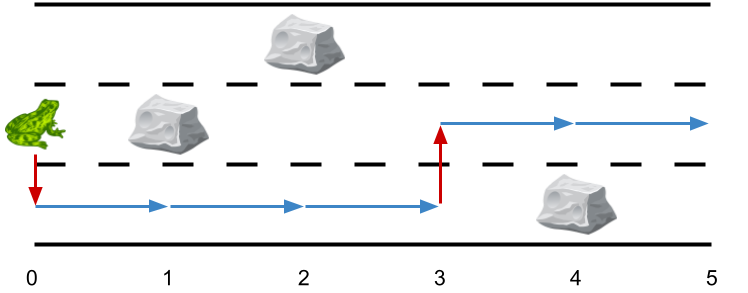1824. Minimum Sideway Jumps

There is a 3 lane road of length `n` that consists of `n + 1` points labeled from `0` to `n`. A frog starts at point `0` in the second lane and wants to jump to point `n`. However, there could be obstacles along the way.

You are given an array `obstacles` of length `n + 1` where each `obstacles[i]` (ranging from 0 to 3) describes an obstacle on the lane `obstacles[i]` at point `i`. If `obstacles[i] == 0`, there are no obstacles at point `i`. There will be at most one obstacle in the 3 lanes at each point.

• For example, if `obstacles == 1`, then there is an obstacle on lane 1 at point 2.

The frog can only travel from point `i` to point `i + 1` on the same lane if there is not an obstacle on the lane at point `i + 1`. To avoid obstacles, the frog can also perform a side jump to jump to another lane (even if they are not adjacent) at the same point if there is no obstacle on the new lane.

• For example, the frog can jump from lane 3 at point 3 to lane 1 at point 3.

Return the minimum number of side jumps the frog needs to reach any lane at point n starting from lane `2` at point 0.

Note: There will be no obstacles on points `0` and `n`.

Example 1:```Input: obstacles = [0,1,2,3,0]
Output: 2
Explanation: The optimal solution is shown by the arrows above. There are 2 side jumps (red arrows).
Note that the frog can jump over obstacles only when making side jumps (as shown at point 2).
```

Example 2:```Input: obstacles = [0,1,1,3,3,0]
Output: 0
Explanation: There are no obstacles on lane 2. No side jumps are required.
```

Example 3:```Input: obstacles = [0,2,1,0,3,0]
Output: 2
Explanation: The optimal solution is shown by the arrows above. There are 2 side jumps.
```

Constraints:

• `obstacles.length == n + 1`
• `1 <= n <= 5 * 105`
• `0 <= obstacles[i] <= 3`
• `obstacles == obstacles[n] == 0`

1824. Minimum Sideway Jumps
``````struct Solution;

impl Solution {
fn min_side_jumps(obstacles: Vec<i32>) -> i32 {
let n = obstacles.len();
let mut dp: Vec<Vec<i32>> = vec![vec![0, 0, 0]; n];
dp = 1;
dp = 1;
for i in 1..n {
for j in 0..3 {
if obstacles[i] == (j + 1) as i32 {
dp[i][j] = std::i32::MAX;
} else {
let mut min = std::i32::MAX;
for k in 0..3 {
if !(obstacles[i - 1] == (k + 1) as i32 || obstacles[i] == (k + 1) as i32) {
min = min.min(dp[i - 1][k] + if k == j { 0 } else { 1 });
}
}
dp[i][j] = min;
}
}
}
*dp[n - 1].iter().min().unwrap()
}
}

#[test]
fn test() {
let obstacles = vec![0, 1, 2, 3, 0];
let res = 2;
assert_eq!(Solution::min_side_jumps(obstacles), res);
let obstacles = vec![0, 1, 1, 3, 3, 0];
let res = 0;
assert_eq!(Solution::min_side_jumps(obstacles), res);
let obstacles = vec![0, 2, 1, 0, 3, 0];
let res = 2;
assert_eq!(Solution::min_side_jumps(obstacles), res);
}
``````